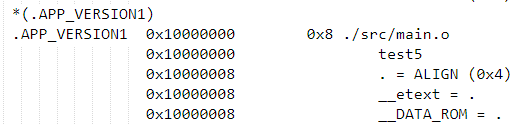# how to use" __attribute__ ((section(""))) "to lnitialize a variable

cancel
Showing results for
Did you mean:
SOLVED

## how to use" __attribute__ ((section(""))) "to lnitialize a variable

4,948 ViewsContributor V

l want to initialize the memory 0x10000000-0x1000 0007 with {0x08,0x07,0x06,0x05,0x04,0x03,0x02,0x01}, I set

in   .c

__attribute__ ((section(".APP_VERSION1"))) uint8_t test5={0x08,0x07,0x06,0x05,0x04,0x03,0x02,0x01} ;

for(i=0;i<8;i++)   {sourceBuffer[i]=test5[i];}

I found from test5 to test5 and sourceBuffer to sourceBuffer is 0xff,who can tell me how to initialize it,thank you for you response.

below is my code

in   .c

__attribute__ ((section(".APP_VERSION1"))) uint8_t test5={0x08,0x07,0x06,0x05,0x04,0x03,0x02,0x01} ;

in .ld

MEMORY
{
/* Flash */
m_interrupts (RX) : ORIGIN = 0x00000000, LENGTH = 0x00000400
m_flash_config (RX) : ORIGIN = 0x00000400, LENGTH = 0x00000010
m_text (RX) : ORIGIN = 0x00000410, LENGTH = 0x0007FBF0-0x8000

/* ccp_1 (RX : ORIGIN = 0x00042000, LENGTH = 0x00002000
name_1 (RX) : ORIGIN = 0x00044000, LENGTH = 0x00002000 */

ccp_1 (RW) : ORIGIN = 0x10000000, LENGTH = 0x00002000
name_1 (RW) : ORIGIN = 0x10002000, LENGTH = 0x00002000

/* SRAM_L */
m_data (RW) : ORIGIN = 0x1FFF8000, LENGTH = 0x00008000 /*//20K*/

/* my_data1 (1FFF8000 1FFF CFFFF )20K ( 1FFFD000 1FFFFFFF 8K )
my_data1 (RW) : ORIGIN = 0x1FFFd000, LENGTH = 0x00002000
*/

/* SRAM_U */
m_data_2 (RW) : ORIGIN = 0x20000000, LENGTH = 0x00007000
}

SECTIONS
{

...

.APP_VERSION1 :
{
. = ALIGN(4);
KEEP (*(.APP_VERSION1))
. = ALIGN(4);
} > ccp_1

...}

Original Attachment has been moved to: flash_TEST.rar

Tags (1)
1 Solution
3,365 ViewsContributor V

hi,Daniel

my purpose is just want to initialize DATA_FLASH section with initialize variable on use

"  __attribute__ ((section(".APP_VERSION1"))) ”,in my project I found the DATA_FLASH is not initialize.

In  CodeWarrior-IDE software,we can use the below code to initialize dataflash

#pragma CONST_SEG APP_VERSION
volatile const __far char AppVersion_ROM=                    {'S','C','R','1','6','_','0','0','2','_','0','1','3','(','2','0','1','7','0','7','2','7',')'};
volatile const __far ubyte Inca_writecode_ROM =1 ; //INCA刷写CODE 0 不支持 1 支持

so I want to set data_flash similar with above code on use" __attribute__ ".

__attribute__ ((section(".APP_VERSION1"))) uint32_t test3={0x01,0x02};
__attribute__ ((section(".APP_VERSION1"))) char AppVersion_ROM=      {'S','C','R','1','6','_','0','0','2','_','0','1','3','(','2','0','1','7','0','7','2','7',')'};

3 Replies
3,365 ViewsNXP TechSupport

Hi,

The code is correct. It works for me at least.

What do you see in the Memory window?And in the .map file?Can you attach you project?

Regards,

Daniel

3,365 ViewsContributor V

Hi ,Daniel

Today, l debug the code, l find use attribute to initialize memory is success, the reason that why the memory data became 0xff is that  l use "FLASH_DRV_DEFlashPartition" function ,it caused data_flash memory data is erased.

so l think,l can use boot and APP code, boot code is used to initialize memory section ,app code is used to initialize dataflash memory data on using "attribute".

whether my thought is right or wrong, please tell me, very thank you. you have helped me a lot.

3,366 ViewsContributor V

hi,Daniel

my purpose is just want to initialize DATA_FLASH section with initialize variable on use

"  __attribute__ ((section(".APP_VERSION1"))) ”,in my project I found the DATA_FLASH is not initialize.

In  CodeWarrior-IDE software,we can use the below code to initialize dataflash

#pragma CONST_SEG APP_VERSION
volatile const __far char AppVersion_ROM=                    {'S','C','R','1','6','_','0','0','2','_','0','1','3','(','2','0','1','7','0','7','2','7',')'};
volatile const __far ubyte Inca_writecode_ROM =1 ; //INCA刷写CODE 0 不支持 1 支持

so I want to set data_flash similar with above code on use" __attribute__ ".

__attribute__ ((section(".APP_VERSION1"))) uint32_t test3={0x01,0x02};
__attribute__ ((section(".APP_VERSION1"))) char AppVersion_ROM=      {'S','C','R','1','6','_','0','0','2','_','0','1','3','(','2','0','1','7','0','7','2','7',')'};